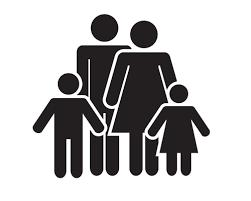# Younger 4782

The son, mother, and father are 96 years old. A mother is 23 years older than her son and five years younger than his father. How old is a son?

s =  15

### Step-by-step explanation:

s+m+o=96
m = 23+s
m = o - 5

m+o+s = 96
m-s = 23
m-o = -5

Row 2 - Row 1 → Row 2
m+o+s = 96
-o-2s = -73
m-o = -5

Row 3 - Row 1 → Row 3
m+o+s = 96
-o-2s = -73
-2o-s = -101

Pivot: Row 2 ↔ Row 3
m+o+s = 96
-2o-s = -101
-o-2s = -73

Row 3 - -1/-2 · Row 2 → Row 3
m+o+s = 96
-2o-s = -101
-1.5s = -22.5

s = -22.5/-1.5 = 15
o = -101+s/-2 = -101+15/-2 = 43
m = 96-o-s = 96-43-15 = 38

m = 38
o = 43
s = 15

Our linear equations calculator calculates it.Did you find an error or inaccuracy? Feel free to write us. Thank you!

Tips for related online calculators
Do you have a linear equation or system of equations and looking for its solution? Or do you have a quadratic equation?
Do you want to convert time units like minutes to seconds?